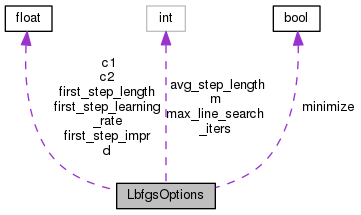LbfgsOptions Struct Reference

This is an implementation of L-BFGS. More...

`#include <optimization.h>`

Collaboration diagram for LbfgsOptions:[legend]

## Public Member Functions

LbfgsOptions (bool minimize=true)

## Public Attributes

bool minimize

int m

float first_step_learning_rate

float first_step_length

float first_step_impr

float c1

float c2

float d

int max_line_search_iters

int avg_step_length

## Detailed Description

This is an implementation of L-BFGS.

It pushes responsibility for determining when to stop, onto the user. There is no call-back here: everything is done via calls to the class itself (see the example in matrix-lib-test.cc). This does not implement constrained L-BFGS, but it will handle constrained problems correctly as long as the function approaches +infinity (or -infinity for maximization problems) when it gets close to the bound of the constraint. In these types of problems, you just let the function value be +infinity for minimization problems, or -infinity for maximization problems, outside these bounds).

Definition at line 84 of file optimization.h.

## ◆ LbfgsOptions()

 LbfgsOptions ( bool minimize = `true` )
inline

Definition at line 107 of file optimization.h.

107  :
108  minimize(minimize),
109  m(10),
111  first_step_length(0.0),
112  first_step_impr(0.0),
113  c1(1.0e-04),
114  c2(0.9),
115  d(2.0),
117  avg_step_length(4) { }
float first_step_learning_rate
Definition: optimization.h:87

## ◆ avg_step_length

 int avg_step_length

Definition at line 104 of file optimization.h.

Referenced by OptimizeLbfgs< Real >::RecordStepLength().

## ◆ c1

 float c1

Definition at line 99 of file optimization.h.

Referenced by OptimizeLbfgs< Real >::StepSizeIteration().

## ◆ c2

 float c2

Definition at line 100 of file optimization.h.

Referenced by OptimizeLbfgs< Real >::StepSizeIteration().

## ◆ d

 float d

Definition at line 101 of file optimization.h.

Referenced by OptimizeLbfgs< Real >::ComputeNewDirection().

## ◆ first_step_impr

 float first_step_impr

Definition at line 94 of file optimization.h.

## ◆ first_step_learning_rate

 float first_step_learning_rate

Definition at line 87 of file optimization.h.

Referenced by OptimizeLbfgs< Real >::ComputeHifNeeded().

## ◆ first_step_length

 float first_step_length

Definition at line 90 of file optimization.h.

## ◆ m

 int m

Definition at line 86 of file optimization.h.

## ◆ max_line_search_iters

 int max_line_search_iters

Definition at line 103 of file optimization.h.

Referenced by OptimizeLbfgs< Real >::StepSizeIteration().

## ◆ minimize

The documentation for this struct was generated from the following file: